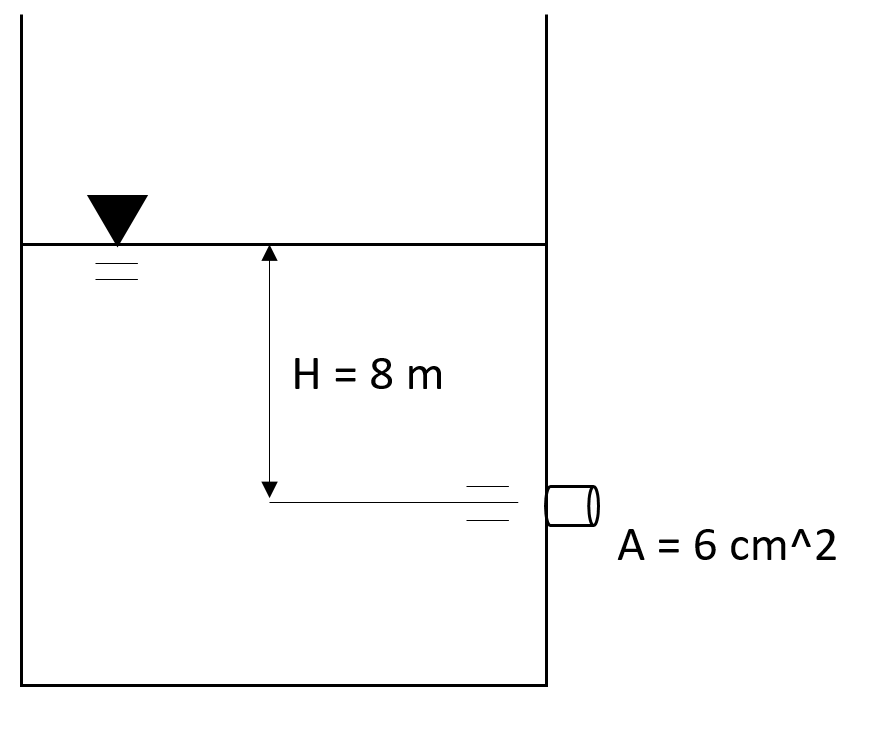## Water Tank

Consider the below water tank with water height H = 8 meters and a nozzle cross-sectional area A = 6 cm^2. Assuming there are no minor losses in the discharge, and a common coefficient of discharge of 0.6, what is the tank’s discharge velocity?Hint
$$Q=CA(2gh)^{1/2}$$$where $$Q$$ is the volumetric flow rate, $$C$$ is the coefficient of discharge, $$A$$ is the cross sectional area of flow, $$g$$ is the acceleration due to gravity, and $$h$$ is the height of the fluid above the orifice. Hint 2 $$Q=vA$$$
where $$Q$$ is the volumetric flow rate, $$v$$ is the fluid’s velocity, and $$A$$ is the cross sectional area of flow.
For an orifice discharging fluid freely into the atmosphere:
$$Q=CA(2gh)^{1/2}$$$where $$Q$$ is the volumetric flow rate, $$C$$ is the coefficient of discharge, $$A$$ is the cross sectional area of flow, $$g$$ is the acceleration due to gravity, and $$h$$ is the height of the fluid above the orifice. Since $$Q=vA$$ where $$v$$ is the fluid’s velocity and $$A$$ is the cross sectional area of flow, we can combine the two equations into one and rearrange to solve for velocity: $$vA=CA(2gh)^{1/2}$$$
$$v=C(2gh)^{1/2}=(0.6)\sqrt{2(9.8m/s^2)(8m)}$$$$$v=(0.6)\sqrt{156.8m^2/s^2)}=(0.6)(12.52m/s)=7.5\:m/s$$$
7.5 m/s